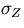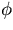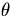Next: Banks in the Event Up: Banks in Begin_Run records Previous: SWIMMER

## SMEARING

The bank is TBCRTEed by subroutine SMRRIN (Run initialization of SMEAR) and stores parameter values used by the SMEAR.

Element# 		: 		 2
TBPUT by 		:		 Subroutine SMRRIN
Contents 		: 		 Smearing job information.
Format 		: 		 R*4 4*NVX+77 variable length where NVX is the number            of VTX layers + 1.



RBUF(1)=seed value for smearing.
(2)=magnetic field ( kG ).
(11-14)=Rmin, Rmax, Zmin, Zmax.
(15)=´# sampling points.
(16)=cut on # sampling points.
(17-18)=t#tex2html_wrap_inline607#,(31-33)=Number of,(barrel) and r (endcap)segments of EM calorimeter.
(34-37)=Rmin, Rmax, Zmin, Zmax.
(38-39)=t#tex2html_wrap_inline625# pedestal and at 1 GeV for (barrel EM).
(40-41)=t#tex2html_wrap_inline627# pedestal and at 1 GeV for (endcap EM).
(51-53)=Number,(barrel), and r(endcap) segmentsof HD calorimeter.
(58-59)=t#tex2html_wrap_inline635# pedestal and at 1 GeV for (barrel HD)
(60-61)=t#tex2html_wrap_inline637# pedestal and at 1 GeV for (endcap HD)
(71)=Number of VTX layers + 1 = NVX
(72-73)=VTX resolution inand z(cm)
(74-76)=r(cm), Z-(cm), Z+(cm)  of beampipe.
(77)=thickness in radiation length of beam pipe.
(78-80)=r(cm), Z-(cm), Z+(cm) of 1st VTX layer.
(81)=thickness in radiation length of 1st VTX layer.
(82-84)=r(cm), Z-(cm), Z+(cm) of 2nd VTX layer.
(85)=thickness in radiation length 2nd VTX layer.
.....
(4*NVX+74)=r(cm) of CDC inner cylinder
(+75)=Z-(cm) of CDC inner cylinder
(+76)=Z+(cm) of CDC inner cylinder
(+77)=thickness in radiation length of CDC inner cylinderNext: Banks in the Event Up: Banks in Begin_Run records Previous: SWIMMER
Miyamoto Akiya
11/20/1998